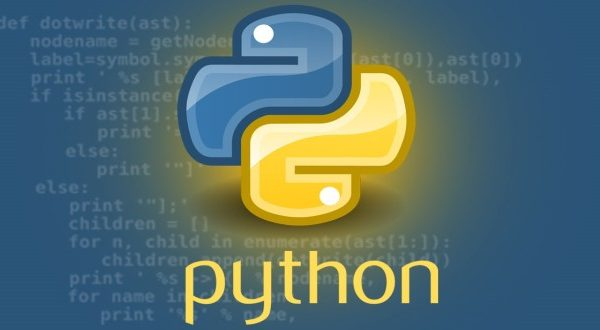# Seaborn Box Plot – Tutorial and Examples### Introduction

Seaborn is one of the most widely used data visualization libraries in Python, as an extension to Matplotlib. It offers a simple, intuitive, yet highly customizable API for data visualization.

In this tutorial, we’ll take a look at how to plot a Box Plot in Seaborn.

Box plots are used to visualize summary statistics of a dataset, displaying attributes of the distribution like the data’s range and distribution.

### Import Data

We’ll need to select a dataset with continuous features in order to create a Box Plot, because Box Plots display summary statistics for continuous variables – the median and range of a dataset. We’ll be working with the Forest Fires dataset.

We’ll begin with importing Pandas to load and parse the dataset. We’ll obviously want to import Seaborn as well. Finally, we’ll import the Pyplot module from Matplotlib, so